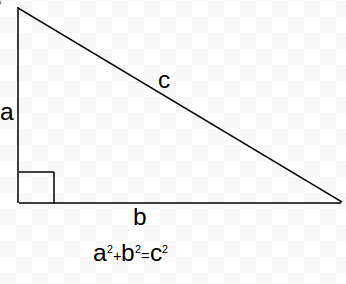Pythagoras theorem

## Objective:

To verify the Pythagoras Theorem

## Pythagoras Theorem

In mathematics, the Pythagorean theorem is a relation in Euclidean geometry among the three sides of a right triangle (right-angled triangle).

In terms of areas, it states: In any right-angled triangle, the area of the square whose side is the hypotenuse (the side opposite the right angle) is equal to the sum of the areas of the squares whose sides are the two legs (the two sides that meet at a right angle).Fig(a)Fig(b)

The theorem can be written as an equation relating the lengths of the sides a, b and c, often called the Pythagorean equation  a2+b2=c2where c represents the length of the hypotenuse, and a and b represent the lengths of the other two sides.

## Example:Given a right angled triangle ABC, length AB = ?

By Pythagoras theorem, we have:
AB2=BC2+CA2
=(2.5)2+(6)2
=42.25
so,                                    AB=6.5m